# Please answer problem #5 thank you str.c #include "str.h" #include <stdio.h> int str_len(...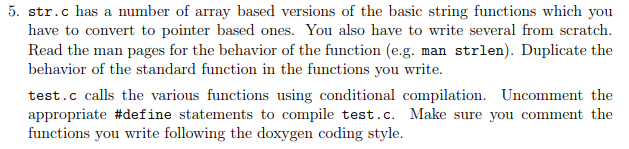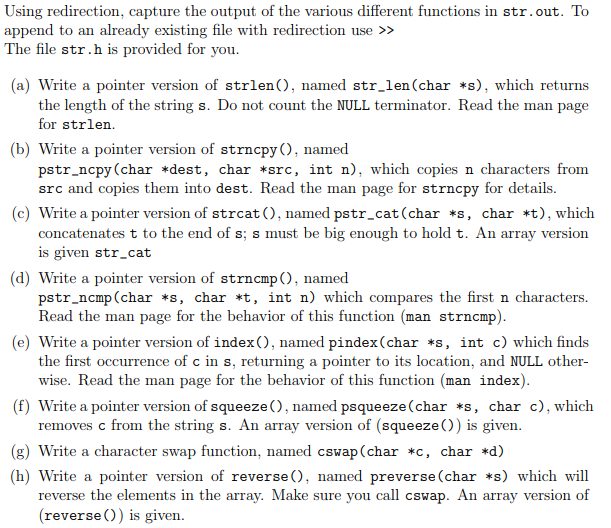str.c

#include "str.h"
#include <stdio.h>

int str_len(char *s)
{
/* this is here so code compiles */
return 0;
}

/* array version */
/* concantenate t to the end of s; s must be big enough */
void str_cat(char s[], char t[])
{
int i, j;

i = j = 0;
while (s[i] != '\0')    /* find end of s */
i++;
while ((s[i++] = t[j++]) != '\0') /* copy t */
;
}

/* array version */
void squeeze(char s[], int c)
{
int i, j;

for (i = j = 0; s[i] != '\0'; i++)
if (s[i] != c)
s[j++] = s[i];

s[j] = '\0';
}

void reverse(char s[])
{
int c, i, j;

for (i = 0, j = str_len(s) - 1; i < j; i++, j-- ) {
/* change this so it calls cswap */
c = s[i];
s[i] = s[j];
s[j] = c;
}

}

/* copy n chars of src into dest */
void pstr_ncpy(char *dest, char *src, int n)
{

}

/* concantenate t to the end of s; s must be big enough! */
void pstr_cat(char *s, char *t)
{

}

and the str.h file is:

#ifndef STR_H_
#define STR_H

void squeeze(char s[], int c);
void psqueeze(char *s, int c);
void str_cat(char s[], char t[]);
void pstr_cat(char *s, char *t);
void pstr_ncpy(char *dest, char *src, int n);
int str_len(char *s);
int pstr_ncmp(char *s, char *t, int n);
void cswap(char *c, char *d);
void preverse(char *s);
char *pindex(char *s, int c);
#endif

and here is test.c file:

#include <stdio.h>
#include "str.h"
#include <stdlib.h>
#include <string.h>

#define STRLEN
/* #define PSTRNCPY */
/* #define PSTRCAT */
/* #define PSTRNCMP */
/* #define PINDEX */
/* #define PSQUEEZE */
/* #define PREVERSE */

#define SIZE 100

int main(void)
{

char s[] = "c run, c run unix, run unix run";

#ifdef STRLEN
printf("the length of \"%s\" is %d\n", s, str_len(s));

#endif

#ifdef PSTRNCPY
char s1[SIZE];

/* copy s into s1 */
pstr_ncpy(s1, s, str_len(s));
printf("s1 = %s\n", s1);
#endif

#ifdef PSTRCAT
char s1[SIZE];
char *s2 = ", go c, go!";

/* copy s into s1 */
pstr_ncpy(s1, s, str_len(s));
printf("s1 = %s\n", s1);
pstr_cat(s1, s2);
printf("s1 = %s\n", s1);
#endif

#ifdef PSTRNCMP
char *t = "look c, look c";
char *s2 = "look c, go c!";
int min;
int cmp;

/* use the smallest length */
min = str_len(s);
if (str_len(t) < min)
min = str_len(t);

printf("comparing:\n");
printf("%s\nand\n", s);
printf("%s\n", t);
if ((cmp = pstr_ncmp(s, t, min))) {
printf("strings differ in the first %d chars; cmp = %d\n", min, cmp);
} else
printf("strings are the same for %d chars; cmp = %d\n", min, cmp);

min = 8;
printf("\ncomparing:\n");
printf("%s\nand\n", t);
printf("%s\n", s2);

if ((cmp =pstr_ncmp(t, s2, min)))
printf("strings differ in the first %d chars; cmp = %d\n", min, cmp);
else
printf("strings are the same for %d chars; cmp = %d\n", min, cmp);

min++;

printf("\ncomparing:\n");
printf("%s\nand\n", t);
printf("%s\n", s2);

if ((cmp =pstr_ncmp(t, s2, min)))
printf("strings differ in the first %d chars; cmp = %d\n", min, cmp);
else
printf("strings are the same for %d chars; cmp = %d\n", min, cmp);

#endif

#ifdef PINDEX

printf("%s\n", s);
char c = 'x';
char *t = pindex(s, c);

printf("searching for %c in \"%s\"\n", c, s);
if (t != NULL)
printf("%c is located at address %p\nwhich is %ld bytes from the base address %p\n", c, t, t - s, s);
else

c = 'a';
t = pindex(s, c);
printf("searching for %c in \"%s\"\n", c, s);
if (t != NULL)
printf("%c is located at address %p\nwhich is %ld bytes from the base address %p\n", c, t, t - s, s);
else
#endif

#ifdef PSQUEEZE

int c = 'r';
printf("%s\n", s);
psqueeze(s, c);
printf("%s\n", s);
#endif

#ifdef PREVERSE

printf("%s\n", s);
printf("reversed is\n");
preverse(s);
printf("%s\n", s);
#endif

return 0;

}

5. str.c has a number of array based versions of the basic string functions which you have to convert to pointer based ones. You also have to write several from scratch Read the man pages for the behavior of the function (e.g. man strlen). Duplicate the behavior of the standard function in the functions you write. test.c calls the various functions using conditional compilation. Uncomment the appropriate #define statements to compile test. C. Make sure you comment the functions you write following the doxygen coding style.
Using redirection, capture the output of the various different functions in str.out. To append to an already existing file with redirection use >> The file str.h is provided for you (a) Write a pointer version of strlen(), named str_len (char *s), which returns the length of the string s. Do not count the NULL terminator. Read the man page for strlen. (b) Write a pointer version of strncpy, named pstr_ncpy(char *dest, char *src, int n), which copies n characters from src and copies them into dest. Read the man page for strncpy for details. (c) Write a pointer version of strcat (), named pstr_cat(char *s, char *t), which concatenates t to the end of s; s must be big enough to hold t. An array version is given str_cat (d) Write a pointer version of strncmp , named pstr_ncmp (char *s, char *t, int n) which compares the first n characters. Read the man page for the behavior of this function (man strn㎝p) e) Write a pointer version of index), named pindex(char *s, int c) which finds the first occurrence of c in s, returning a pointer to its location, and NULL other- wise. Read the man page for the behavior of this function (man index) (f) Write a pointer version of squeeze), named psqueeze(char *s, char c), which removes c from the string s. An array version of (squeeze)) is given (g) Write a character swap function, named cswap(char *c, char *d) (h) Write a pointer version of reverse, named preverse (char *s) which will reverse the elements in the array. Make sure you call cswap. An array version of (reverse)) is given.

// str.h

#ifndef STR_H_

#define STR_H_

void squeeze(char s[], int c);

void psqueeze(char *s, int c);

void str_cat(char s[], char t[]);

void pstr_cat(char *s, char *t);

void pstr_ncpy(char *dest, char *src, int n);

int str_len(char *s);

int pstr_ncmp(char *s, char *t, int n);

void cswap(char *c, char *d);

void preverse(char *s);

char *pindex(char *s, int c);

#endif

//end of str.h

// str.c

#include "str.h"

#include <stdio.h>

#include <stdlib.h>

int str_len(char *s)

{

/* this is here so code compiles */

int count=0;

for(int i=0;s[i]!='\0';i++)

count++;

return count;

}

/* array version */

/* concantenate t to the end of s; s must be big enough */

void str_cat(char s[], char t[])

{

int i, j;

i = j = 0;

while (s[i] != '\0')    /* find end of s */

i++;

while ((s[i++] = t[j++]) != '\0') /* copy t */

;

}

/* array version */

void squeeze(char s[], int c)

{

int i, j;

for (i = j = 0; s[i] != '\0'; i++)

if (s[i] != c)

s[j++] = s[i];

s[j] = '\0';

}

void reverse(char s[])

{

int c, i, j;

for (i = 0, j = str_len(s) - 1; i < j; i++, j-- ) {

/* change this so it calls cswap */

c = s[i];

s[i] = s[j];

s[j] = c;

}

}

/* copy n chars of src into dest */

void pstr_ncpy(char *dest, char *src, int n)

{

for(int i=0;i<n;i++)

*(dest+i) = *(src+i);

*(dest+n) = '\0';

}

/* concantenate t to the end of s; s must be big enough! */

void pstr_cat(char *s, char *t)

{

int i,j;

for(i=0;*(s+i)!='\0';i++);

for(j=0;*(t+j)!='\0';j++,i++)

*(s+i) = *(t+j);

*(s+i) = '\0';

}

int pstr_ncmp(char *s, char *t, int n)

{

for(int i=0;i<n;i++)

{

if(*(s+i) < *(t+i))

return -1;

else if(*(s+i) > *(t+i))

return 1;

}

return 0;

}

char *pindex(char *s, int c)

{

for(int i=0;*(s+i)!= '\0';i++)

if(*(s+i) == c)

return (s+i);

return NULL;

}

void psqueeze(char *s, int c)

{

int i, j;

for (i = j = 0; s[i] != '\0'; i++)

if (*(s+i) != c)

{

*(s+j) = *(s+i);

j++;

}

*(s+j) = '\0';

}

void cswap(char *c, char *d)

{

char temp = *c;

*c = *d;

*d = temp;

}

void preverse(char *s)

{

for(int i=0,j=str_len(s)-1;i<str_len(s)/2;i++,j--)

{

cswap((s+i),(s+j));

}

}

//end of str.c

// test.c

#include <stdio.h>

#include "str.h"

#include <stdlib.h>

#include <string.h>

/* #define STRLEN */

/* #define PSTRNCPY */

/* #define PSTRCAT */

/* #define PSTRNCMP */

/* #define PINDEX */

/* #define PSQUEEZE */

/* #define PREVERSE */

#define SIZE 100

int main(void)

{

char s[] = "c run, c run unix, run unix run";

#ifdef STRLEN

printf("the length of \"%s\" is %d\n", s, str_len(s));

#endif

#ifdef PSTRNCPY

char s1[SIZE];

/* copy s into s1 */

pstr_ncpy(s1, s, str_len(s));

printf("s1 = %s\n", s1);

#endif

#ifdef PSTRCAT

char s1[SIZE];

char *s2 = ", go c, go!";

/* copy s into s1 */

pstr_ncpy(s1, s, str_len(s));

printf("s1 = %s\n", s1);

pstr_cat(s1, s2);

printf("s1 = %s\n", s1);

#endif

#ifdef PSTRNCMP

char *t = "look c, look c";

char *s2 = "look c, go c!";

int min;

int cmp;

/* use the smallest length */

min = str_len(s);

if (str_len(t) < min)

min = str_len(t);

printf("comparing:\n");

printf("%s\nand\n", s);

printf("%s\n", t);

if ((cmp = pstr_ncmp(s, t, min))) {

printf("strings differ in the first %d chars; cmp = %d\n", min, cmp);

} else

printf("strings are the same for %d chars; cmp = %d\n", min, cmp);

min = 8;

printf("\ncomparing:\n");

printf("%s\nand\n", t);

printf("%s\n", s2);

if ((cmp =pstr_ncmp(t, s2, min)))

printf("strings differ in the first %d chars; cmp = %d\n", min, cmp);

else

printf("strings are the same for %d chars; cmp = %d\n", min, cmp);

min++;

printf("\ncomparing:\n");

printf("%s\nand\n", t);

printf("%s\n", s2);

if ((cmp =pstr_ncmp(t, s2, min)))

printf("strings differ in the first %d chars; cmp = %d\n", min, cmp);

else

printf("strings are the same for %d chars; cmp = %d\n", min, cmp);

#endif

#ifdef PINDEX

printf("%s\n", s);

char c = 'x';

char *t = pindex(s, c);

printf("searching for %c in \"%s\"\n", c, s);

if (t != NULL)

printf("%c is located at address %p\nwhich is %ld bytes from the base address %p\n", c, t, t - s, s);

else

c = 'a';

t = pindex(s, c);

printf("searching for %c in \"%s\"\n", c, s);

if (t != NULL)

printf("%c is located at address %p\nwhich is %ld bytes from the base address %p\n", c, t, t - s, s);

else

#endif

#ifdef PSQUEEZE

int c = 'r';

printf("%s\n", s);

psqueeze(s, c);

printf("%s\n", s);

#endif

#ifdef PREVERSE

printf("%s\n", s);

printf("reversed is\n");

preverse(s);

printf("%s\n", s);

#endif

return 0;

}

//end of test.c

Output: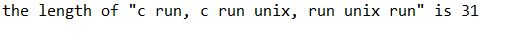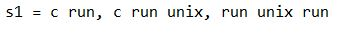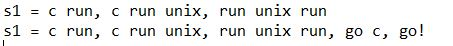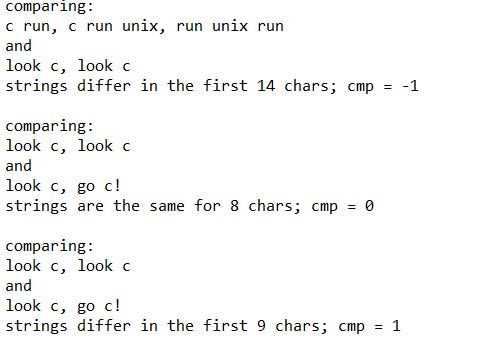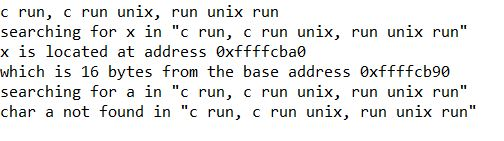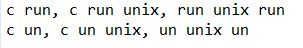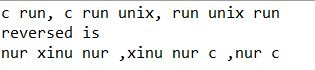More Homework Help Questions Additional questions in this topic.